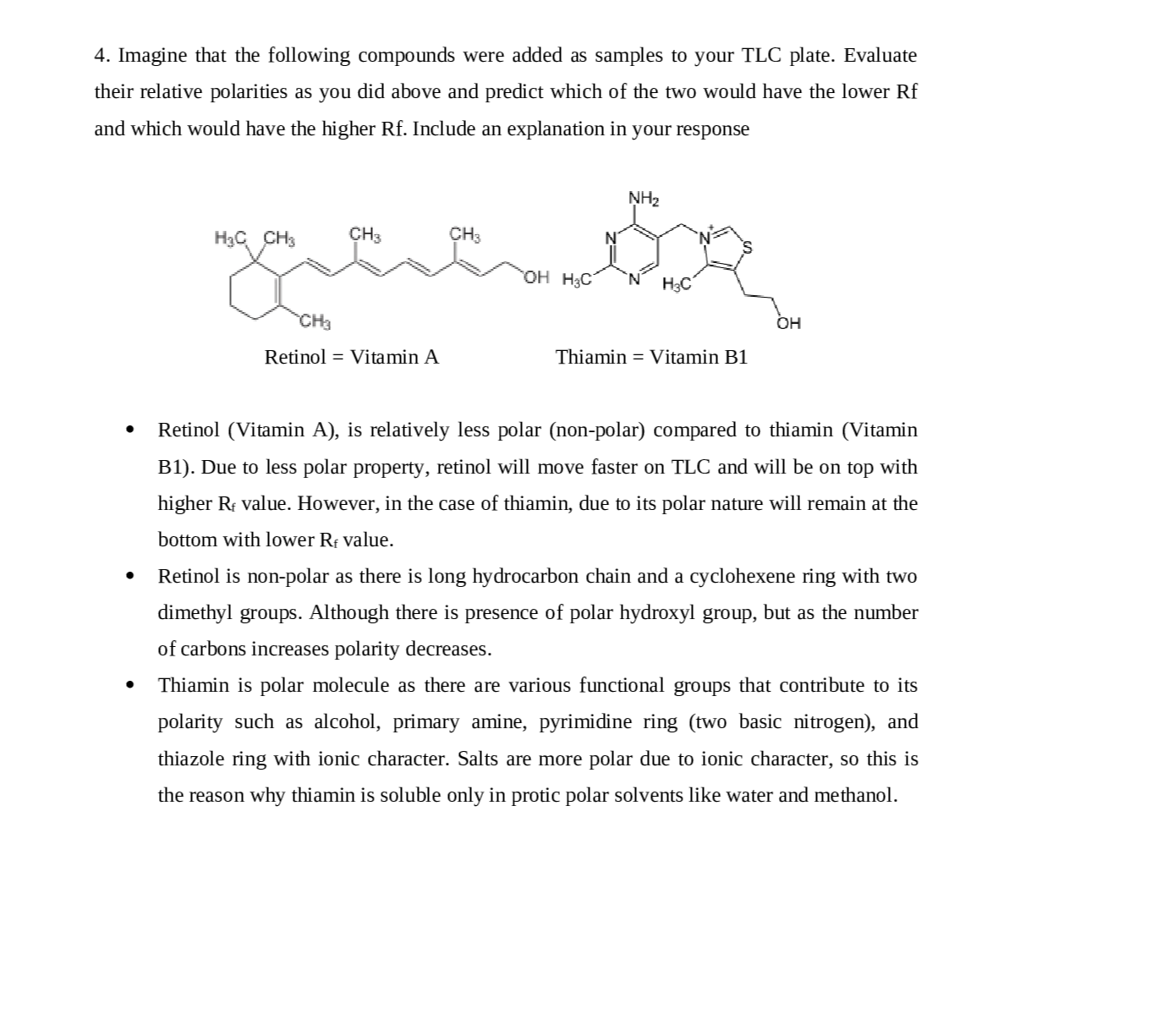Question

Analytical Chemistry

4. (2 pts.) Imagine that the following compounds were added as samples to your TLC plate. Evaluate their relative polarities as you did above and predict which of the two would have the lower Rf and which would have the higher Rf. Include an explanation in your responseVerified### Question 43728Analytical Chemistry

4.The formation of C2H;CI(g) is an exothermic reaction (AH° = –72.6 kJ/molpxn). Thefollowing two step reaction mechanism is proposed:
Step 1:C,H4(g) + HCI(g) → C,H;*(g) + C-(g)Slow step
Step 2: C,H;*(g) + Cl-(g) → C;H;CI(g)Fast step
A.) Write the rate law for the reaction that is consistent with the reaction mechanism above.
B.) Identify an intermediate in the reaction mechanism
above. Using the axes provided, draw a curve that shows energy changes that occur during the progress of the reaction. The curve should illustrate both proposed two-step mechanisms and the enthalpy change of the reaction.

### Question 43727Analytical Chemistry

The two reaction diagrams here represent the same reaction: one without a catalyst an done with a catalyst. Estimate the activation energy for each process, and identify which one involves a catalyst.13.

### Question 43726Analytical Chemistry

Consider the four reaction-energy diagrams below:
Identify the two diagrams that could represent a catalyzed and an un catalyzed reaction pathway for the same reaction. Indicate which of the two diagrams represents the catalyzed reaction pathway for the reaction.

### Question 43725Analytical Chemistry

Nitrogen dioxide, NO2(g), is produced as a by-product of the combustion of fossil fuels ininternal combustion engines. At elevated temperatures NO2(g) decomposes according to theequation below.11.
2 \mathrm{NO}_{2}(g) \rightarrow 2 \mathrm{NO}(g)+\mathrm{O}_{2}(g)
The concentration of a sample of NO2(g) is monitored as it decomposes and is recorded onthe graph directly below. The two graphs that follow it are derived from the original data.
A.) What order is the following reaction?
B.) Write the rate law for the decomposition of NO2(g).
C.) Consider two possible mechanisms for the decomposition reaction.
i.) Is the rate law described by the mechanism I shown below consistent with therate law you wrote in part (b)? Justify your answer.
Step 1: NO2(g) + NO2(g) → NO(g) + NO3(g)slow
Step 2: NO3(g) → NO(g) + O2(g)fast
ii.) Is the rate law described by mechanism II shown below consistent with the rate law you wrote in part (b) ? Justify your answer.
Step 1:NO2(g) + NO2(g)N„O4(g)fast equilibrium
Step 2:N204(9) → 2 NO(g) + O2(g)slow

### Question 43724Analytical Chemistry

What is the expected rate law for the overall reaction shown below?
Step 1:NO(g) + Br,(g) 2 NOBr2(g)(fast)
Step 2:NOBR,(g) + NO2 NO (g) + 2 Br(g)(slow)

### Question 43723Analytical Chemistry

2NO2(g) + F2(g) →
NO, and F2 can react to produce NO,F as represented above. A proposed mechanism for this reaction has two elementary steps, as shown below.reaction that is consistent with the proposed mechanism.Write a rate law for the overall
NO, + F, → NO,F + F(slow)
NO2 + FNO,F(fast)

### Question 43722Analytical Chemistry

What is the rate law for the overall reaction that is consistent with the proposed mechanism?
HOBR,(g) + HBr(g) –2 НОBr(q)(FAST)
HBr(g)+ O2(g)НОBr:(g)(SLOW)
2 HOBR(g) → H2O2(g) + Br2(g)(FAST)

### Question 43721Analytical Chemistry

\mathrm{Na}_{2} \mathrm{C}_{37} \mathrm{H}_{34} \mathrm{~N}_{2} \mathrm{~S}_{3} \mathrm{O}_{9}+\mathrm{OCl}^{-} \rightarrow \text { products }
Blue food coloring can be oxidized by household bleach (which contains OCI-) to form colorless products, as represented by the equation above. A student used a spectrophotometer set at a wavelength of 635 nm to study the absorbance of the food coloring over time during the bleaching process. In the study, bleach is present in large excess so that the concentration of OCl- is essentially constant throughout the reaction.The students use data from the study to generate the graphs below.

### Question 43720Analytical Chemistry

5. Hydrogen reacts with nitrogen monoxide to form dinitrogen monoxide (laughing gas)according to the equation:
\mathrm{H}_{2}(g)+2 \mathrm{NO}(g) \rightarrow \mathrm{N}_{2} \mathrm{O}(g)+\mathrm{H}_{2} \mathrm{O}(g)
Determine the rate law, the rate constant, and the orders with respect to each reactant from the following data:

### Question 43719Analytical Chemistry

. Ozone in the upper atmosphere is depleted when it reacts with nitrogen oxides. The rates of the reactions of nitrogen oxides with ozone are important factors in deciding how significant these reactions are in the formation of the ozone hole over Antarctica. One such reaction is the combination of nitric oxide, NO, with ozone, O3:
\mathrm{NO}(g)+\mathrm{O}_{3}(g) \rightarrow \mathrm{NO}_{2}(g)+\mathrm{O}_{2}(g)
Use the data to determine the rate law and the rate constant for the reaction at 25°C.

### Submit query